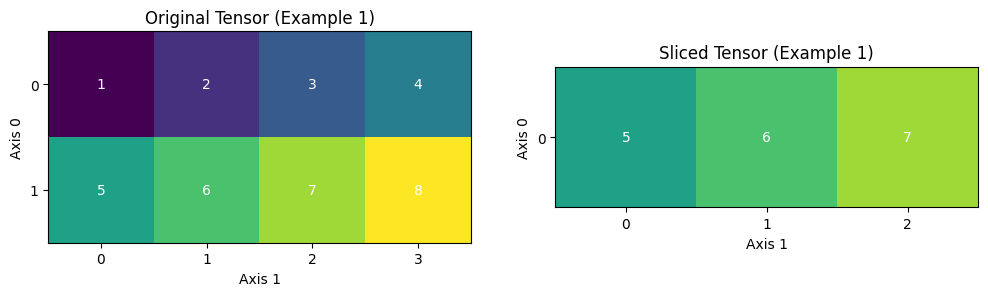# 调试经验¶

## 一、错误日志怎么看¶)

• 原生 Python 的报错栈（对应上图 ①）

• 动转静报错栈起始标志位（对应 ②）

• 实际出错的转写代码位置（对应 ③）

• 静态图相关的框架层面报错信息（对应 ④）

## 二、快速确定问题原因¶

### 2.1 (NotFound) Input("X")¶

```RuntimeError: (NotFound) Input("Filter") of ConvOp should not be null.
[Hint: Expected ctx->HasInputs("Filter") == true, but received ctx->HasInputs("Filter"):0 != true:1.]
[operator < conv2d > error]
```

• 首先确认代码所在的 sublayer 是否继承了 `nn.Layer`

• 此行代码所在函数是否绕开了 foward 函数，单独调用的（2.1 版本之前）

• 如何查看是 Tensor 还是 Vaiable 类型，可以通过 pdb 交互式调试

### 2.2 Expected input_dims[i] == input_dims¶

```[Hint: Expected input_dims[i] == input_dims, but received input_dims[i]:-1, -1 != input_dims:16, -1.]
[operator < xxx_op > error]
```

• 代码层面，判断是否是上游使用了`reshape`导致 -1 的污染性传播

• 可以结合后续调试技巧，判断是否是某个 API 的输出 shape 在动静态图下有 diff 行为

### 2.3 desc->CheckGuards() == true¶

```[Hint: Expected desc->CheckGuards() == true, but received desc->CheckGuards():0 != true: 1.]
```• 模型代码是否存在上述复杂的 Tensor slice 切片操作

• 推荐使用 paddle.slice 接口替换复杂的 Tensor slice 操作

### 2.4 Segment Fault¶

• 每个sublayer 是否继承了 `nn.Layer`

### 2.5 Container 的使用建议¶

• ParameterList

```class MyLayer(paddle.nn.Layer):
def __init__(self, num_stacked_param):
super(MyLayer, self).__init__()

# 此用法下，MyLayer.parameters() 返回为空
self.params = [w1, w2]                            # <----- 错误用法

self.params = paddle.nn.ParameterList([w1, w2])   # <----- 正确用法
```
• LayerList

```class MyLayer(paddle.nn.Layer):
def __init__(self):
super(MyLayer, self).__init__()